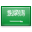# Real-valued functionMass measured in grams is a function from this collection of weight to positive real numbers. The term "weight function", an allusion to this example, is used in pure and applied mathematics.

In mathematics, a real-valued function is a function whose values are real numbers. In other words, it is a function that assigns a real number to each member of its domain.

Many important function spaces are defined to consist of real-valued functions.

## In general

Let X be an arbitrary set. Let ${displaystyle {mathcal {F}}(X,{mathbb {R} })}$denote the set of all functions from X to real numbers R. Because R is a field${displaystyle {mathcal {F}}(X,{mathbb {R} })}$may be turned into a vector space and a commutative algebra over reals by adding the appropriate structure:

• ${displaystyle f+g:xmapsto f(x)+g(x)}$– vector addition
• ${displaystyle mathbf {0} :xmapsto 0}$– additive identity
• ${displaystyle cf:xmapsto cf(x),quad cin {mathbb {R} }}$– scalar multiplication
• ${displaystyle fg:xmapsto f(x)g(x)}$– pointwise multiplication

Also, since R is an ordered set, there is a partial order on ${displaystyle {mathcal {F}}(X,{mathbb {R} })}$:

• ${displaystyle fleq gquad iff quad forall x:f(x)leq g(x)}$.

${displaystyle {mathcal {F}}(X,{mathbb {R} })}$is a partially ordered ring.

## Measurable

The σ-algebra of Borel sets is an important structure on real numbers. If X has its σ-algebra and a function f is such that the preimagef −1(B) of any Borel set B belongs to that σ-algebra, then f is said to be measurable. Measurable functions also form a vector space and an algebra as explained above.

Moreover, a set (family) of real-valued functions on X can actually define a σ-algebra on X generated by all preimages of all Borel sets (or of intervals only, it is not important). This is the way how σ-algebras arise in (Kolmogorov'sprobability theory, where real-valued functions on the sample space Ω are real-valued random variables.

## Continuous

Real numbers form a topological space and a complete metric spaceContinuous real-valued functions (which implies that X is a topological space) are important in theories of topological spaces and of metric spaces. The extreme value theorem states that for any real continuous function on a compact space its global maximum and minimum exist.

The concept of metric space itself is defined with a real-valued function of two variables, the metric, which is continuous. The space of continuous functions on a compact Hausdorff space has a particular importance. Convergent sequences also can be considered as real-valued continuous functions on a special topological space.

Continuous functions also form a vector space and an algebra as explained above, and are a subclass of measurable functions because any topological space has the σ-algebra generated by open (or closed) sets.

## Smooth

Real numbers are used as the codomain to define smooth functions. A domain of a real smooth function can be the real coordinate space (which yields a real multivariable function), a topological vector space, an open subset of them, or a smooth manifold.

Spaces of smooth functions also are vector spaces and algebras as explained above, and are a subclass of continuous functions.

## Appearances in measure theory

measure on a set is a non-negative real-valued functional on a σ-algebra of subsets. Lp spaces on sets with a measure are defined from aforementioned real-valued measurable functions, although they are actually quotient spaces. More precisely, whereas a function satisfying an appropriate summability condition defines an element of Lp space, in the opposite direction for any f ∈ Lp(X) and x ∈ X which is not an atom, the value f(x) is undefined. Though, real-valued Lp spaces still have some of the structure explicated above. Each of Lp spaces is a vector space and have a partial order, and there exists a pointwise multiplication of "functions" which changes p, namely

${displaystyle cdot :L^{1/alpha } imes L^{1/eta } o L^{1/(alpha +eta )},quad 0leq alpha ,eta leq 1,quad alpha +eta leq 1.}$For example, pointwise product of two L2 functions belongs to L1.

## Other appearances

Other contexts where real-valued functions and their special properties are used include monotonic functions (on ordered sets), convex functions (on vector and affine spaces), harmonic and subharmonic functions (on Riemannian manifolds), analytic functions (usually of one or more real variables), algebraic functions (on real algebraic varieties), and polynomials (of one or more real variables).

### أهلاً ومرحباً بكم## قسم الرياضيات### التوقيت والتقويم

```_time.start(["thetimenow"]);
```

```
```

```

```

### محرك بحث جوجل```

```

### للتواصل1. الهاتف : 0164044771

تحويلة: 4771

(QR Code)

mailto:mm.mousa@mu.edu.sa### إعلانات1- الاختبار الفصلى الثانى لمقرر التحليل العددى (يوم الاحد الموافق 3 / 7/ 1440 هـ)

2- الاختبار الفصلى الثانى لمقررحساب المتجهات (يوم الثلاثاء الموافق 5 / 7 / 1440 هـ)

### الساعات المكتبيةالأثنين: 10 - 12

الثلاثاء: 8 - 10

الأربعاء: 8 - 10

### بعض الخدمات الإلكترونية لعمادة تقنية المعلومات### آلة حاسبة

```

```

### التقويم الجامعى### بعض الجوائز والتكريمات### إحصائية الموقع

عدد الصفحات: 258

البحوث والمحاضرات: 155

الزيارات: 101284